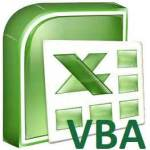# Excel MRound Function round to nearest multipleThis tutorial explains how to use Excel MRound Function round to nearest multiple Excel MRound Function round to nearest multiple Excel Round Function is used to round to nearest digit, while Excel MRound Function is used to round to nearest multiple. The first time I used MRound Function is to round employee bonus to nearest \$100, because we don't want the bonus amount looks better. For example, \$11040 is rounded to \$11000. If you fail to use Excel MRound Function, navigate to Developer &g...
More

# Excel Date FunctionThis Excel tutorial explains how to use Excel Date Function. You may also want to read: Excel first date of month and last date of month Excel Date Function Excel Date function is used to transform year, month, day into Date Serial, a numeric value that represents the Date. Why do you need Excel Date Function? Date is being treated specially in Excel. The format you see in Excel worksheet such as dd/mm/yyyy is only a displayed formatting, the underlying value of a Date is a numeric v...
More

# Excel Date Time ConversionExcel Date Time Conversion Excel has a special way to treat Date Time value but most people are not aware of that. You need to understand the meaning of the numeric values that represent Excel Date Time in order that you can freely perform Excel date time conversion to hours, minutes, days,  etc. After you understand the meaning of the numerical values, you can easily calculate Excel Date Time Difference. Also read the below post about calculate time difference Excel time difference in ho...
More

# Function, Sub, Method, Property DifferencesThis tutorial explains differences among Function, Sub, Method, Property Excel / Access VBA. You may also want to read: Difference between Dim and Set Excel VBA Option Explicit to force declaration of variables Difference between ByRef ByVal Function, Sub, Method, Property Differences In my blog, I have categorized different VBA topics by their properties. If you don't understand Function, Sub, Method, Property Differences, you will find my tips confusing and difficult to use. Thos...
More